# Survey123 Tricks of the Trade: Calculation modes

286
2
2 weeks agobyEsri Notable Contributor
2 2 286

In a Survey123 form, you can automatically set the value of a question using an XLSForm expression in the calculation column. In the following example, the asset condition value is automatically calculated based on a checklist. If the three elements in the checklist are ticked, the condition is set to Good, otherwise the value is set to Needs repair.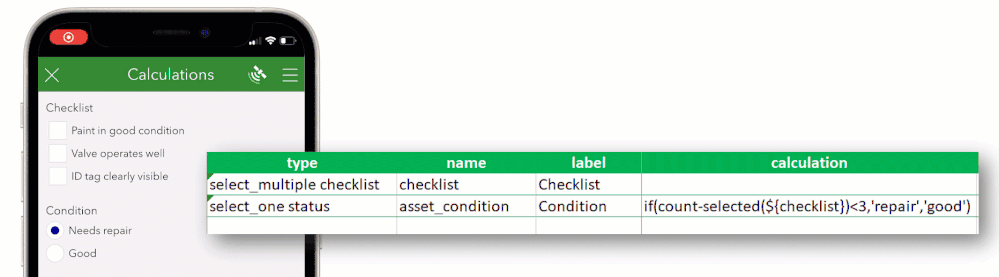Calculations are used for different purposes. Sometimes, we want to save the end user from answering questions for which we already know the answer. For example, the age of a person can be automatically calculated if we already know the birth date.  If we are given the length of a pipe and the price per meter, we can also calculate the total price of the pipe.

In other occasions, we use calculations to provide a best guess, which the user can override.

This blog post introduces the concept of calculation modes. They will allow you to better control when  calculations are evaluated.

### Calculation mode: auto

Unless specified otherwise, calculations are always executed in auto mode. When in auto mode, calculation expressions are evaluated when any of the inputs in the calculation expression change. For example, say you write a calculation to convert a temperature value from Fahrenheit to Celsius. Whenever the Fahrenheit value changes, the Celsius temperature is calculated.Calculations in auto mode will not overwrite a value entered by the end user. For example, if the end user manually enters a value in the calculated question, the temperature conversion will be suspended. Note in the animation below how the Celsius temperature stops being automatically calculated as soon as its value is manually set to 34.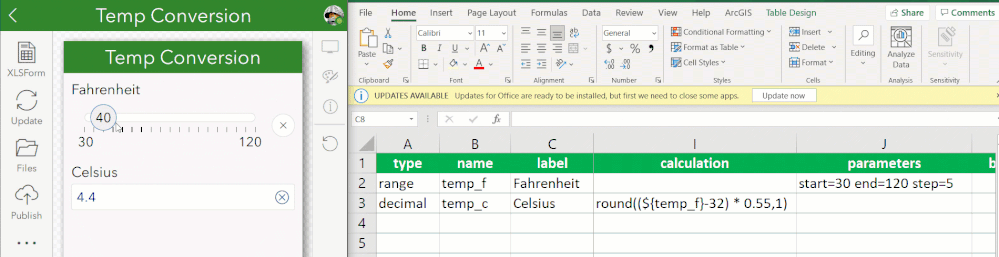While the calculation is suspended, Survey123 will display a Calculate icon on the side to allow the end user to manually trigger the calculation. Only if the end user taps on that button to run the calculation, the expression will be evaluated again.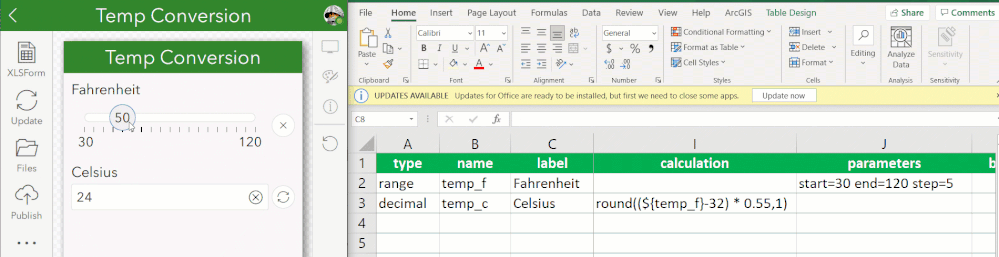Calculations in auto mode are also suspended when an existing record is loaded into a survey. That is, when you open a survey from the Inbox, or when you load a record into the web app to edit it. This is because, again, in auto mode we do not want to overwrite a value that may have been manually added by the user.

In the vast majority of cases, you will find the auto calculation mode fit well your workflow. You do not need to do anything special to set your calculation in auto mode: Add an expression into the calculation mode, and you are set!

If you want to set the calculation mode explicitly, go into the bind::esri:parameters column, and add calculationMode=auto, as shown in the next screenshot.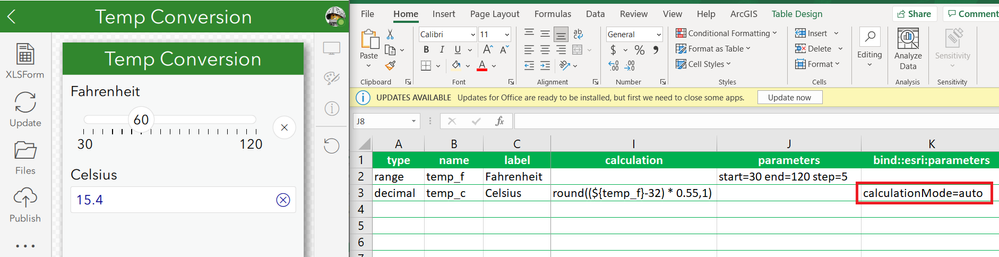The auto calculation mode is supported in both the field and web apps.

### Calculation mode: manual

This one is easy. The question will only calculate when the Calculate button next to the question is pressed.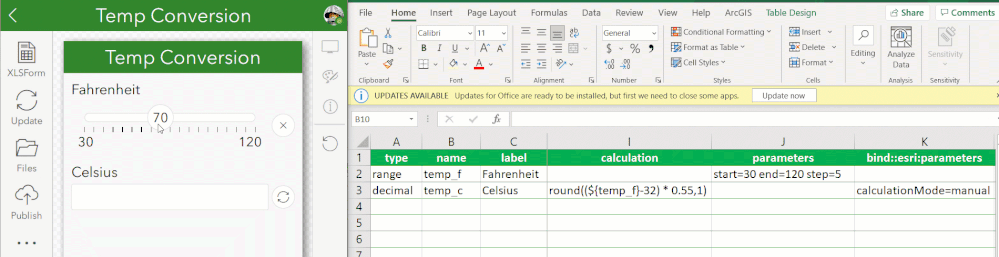This calculation mode is only supported in the field app. The Survey123 web app does not support it.

### Calculation mode: always

Set the calculationMode to always, and your calculation will never be suspended. It will run always, no exceptions. If the user manually resets the calculated value, as soon as any referenced variable in the calculation changes, the expression will be evaluated.The calculation will also be triggered when the form is submitted, just in case!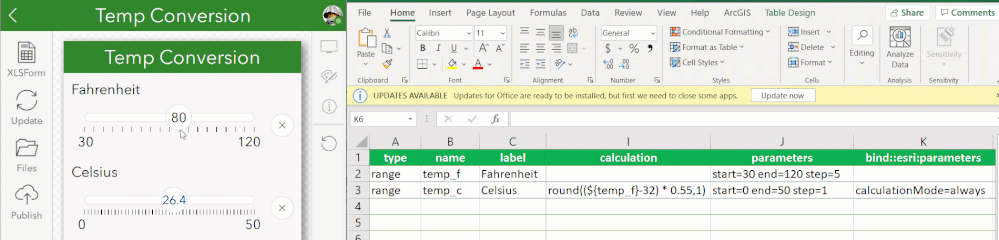I find calculationMode=always particularly useful when working with the Inbox. Lets take the example in the screenshot below: Row 6 is using calculationMode=always because we want its value to be always calculated, even if the record is opened in edit mode.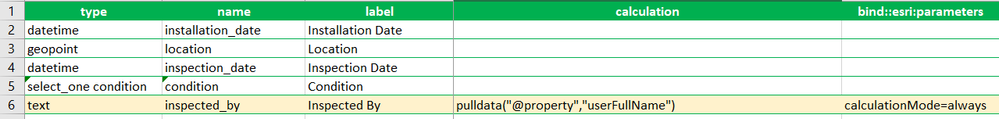In the example above, if you keep the calculationMode as auto, the inspected_by value would remain unchanged if you open the record from the Inbox.

Here you have another variation of the survey: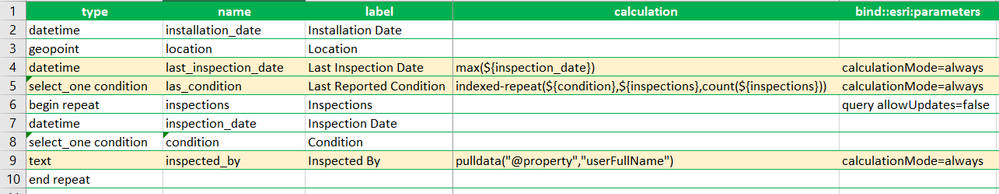In this case, the survey uses a repeat, so you can have information about the asset, and a history of all inspections performed. We use calculationMode=always to keep the last inspection date and last known condition up to date.  Both of these values use data from the inspections table. Again, calculationMode=always helps us make sure these values are refreshed even if he record is loaded from the Inbox.

This calculation mode is only supported in the field app. The Survey123 web app does not support it.

### Calculation mode: whenEmpty

As you may expect, calculationMode=whenEmpty will only trigger the calculation when the target question is empty.  If you are creating a new record or editing it, makes no difference: this calculation mode will be triggered if the question is empty.  Once a nonempty value is returned, the question behaves in the same way as the manual mode.

Check row 7 in the screenshot below. In this case we use calculationMode=whenEmpty to populate the inspection date with the current time, but only if the value is empty. That is, if you are opening an inspection record from the past, the value will not be overwritten, but if you are creating a new inspection record, the value will be automatically populated.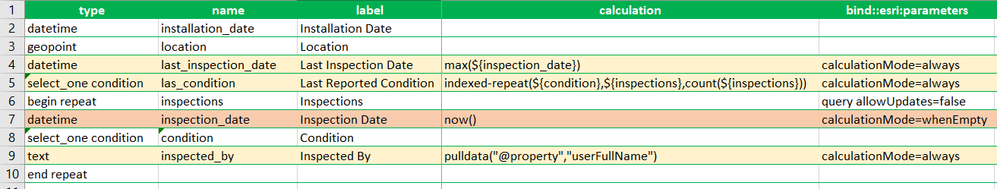This calculation mode is only supported in the field app. The Survey123 web app does not support it.

### Force calculations in the Survey123 web app

The Survey123 web application, at the time when I write this, only supports the auto calculation mode. However, if you happen to be using the Survey123 web app to edit a record, and you want certain calculations to be triggered when the record is loaded, you can.

The forceRecalculate url parameter allows you to specify which questions should be recalculated when the form is opened in edit mode.  If you are not familiar with web app url parameters, check this blog.

For example: ?recalculate=inspector,inspection_date will trigger calculations, and overwrite existing values, for the inspector and inspection_date questions.

You can also use ?recalculate=true to trigger all calculations in the form when the form loads in edit mode.